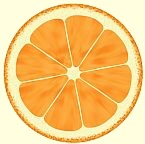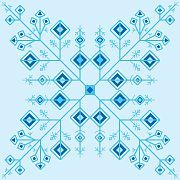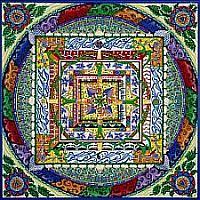Example of Factor Group ConstructionLet Z stand for the set of all integers; 5Z stand for all multiples of five; and 15Z for all multiples of fifteen.

The set Z along with the binary operation of addition forms a group. The proper notation is [Z, +] but it's commonly understood by Z alone.

We can also have groups composed of other things, like maps and matrices, dimensional hierarchies (homology groups) and geometric shapes. With the latter, we're looking for closed sub-symmetries embedded in larger symmetries.

The set 5Z under the operation of addition is a normal subgroup of Z as well as the identity equivalence class of the factor group -- Z/5Z. As a group itself it also has subgroups of its own -- 15Z -- for instance.If we imagine all multiples of five spread out on a table, and below them all multiples of fifteen, we could draw lines from entries in the set of 5Z to corresponding ones in the set of 15Z. The numbers in the set of 5Z left out of the mapping process can then be organized into respective classes according to the rules of arithmetic and the axioms of groups. By so doing we generate the factor group -- 5Z/15Z which is isomorphic to Z3. By the way, Z/5Z is isomorphic to Z5.

Z3 is the group composed of the numbers {0,1,2} under the operation addition. The elements of 5Z map to corresponding elements in Z3, filtered through 15Z. To be precise: zero maps to zero, 5 maps to 1, 10 maps to 2, and then the cycle repeats. Fifteen maps to 0, 20 maps to 1, 25 maps to 2, and so forth. Consequently, all integral multiples of 15 map to zero in Z3. In this way we rearrange our multiples of five into three equivalence classes whose symmetry corresponds -- is congruent to -- that of Z3.

They look like: (..., -30, -15, 0, 15, 30, ...) the identity class which maps to zero in Z3, (..., -40, -25, -10, 5, 20, 35, ...) which maps to 1, and (..., -35, -20, -5, 10, 25, 40,...) which maps to 2. The combined member-numbers of these classes account for all integral multiples of five in Z.

Addition is by representatives from a class.

For example: (-10+30=20), (-25+35=10), (-40+25=-15), and (-20+10=-10). Analogous computations in Z3 are, respectively: 1+0=1, 1+1=2, 1+2=0, and 2+2=1.

And the addition of any representatives from these classes will result in the same class.

For example: (-10+30-20=0) falls into the class identified with 0 in Z3, as do the results of (-25+15-35=-45) and (35+45-20=60). They all correspond to 1+0+2=0.15Z is not only seen to be the identity element of the factor group -- 5Z/15Z -- but also, in the act of factoring, takes on the additional role as kernel of the homomorphism (structure-preserving map) which assigns numbers to respective sets. The kernel generates the equivalence class composed of multiples of 15 and by so doing establishes how numbers are to be distributed -- by the difference of fifteen -- and partitioned into classes -- the elements of the factor group. The kernel sets the pattern, the stamp, the lay of the land, and the constant of difference, in this particular case.

In a nutshell: This normal subgroup/identity equivalence class/kernel of homomorphism -- 15Z -- is mapped to zero -- our additive identity element -- in Z3 under the isomorphism.

And as the factor group -- 5Z/15Z -- is a homomorphic image of 5Z, the operation of addition remains invariant as does the symmetric relationship among parts -- how it's structured. In this manner you can find all homomorphic images of 5Z without going outside the group itself.

Printer Friendly Version [black & white]